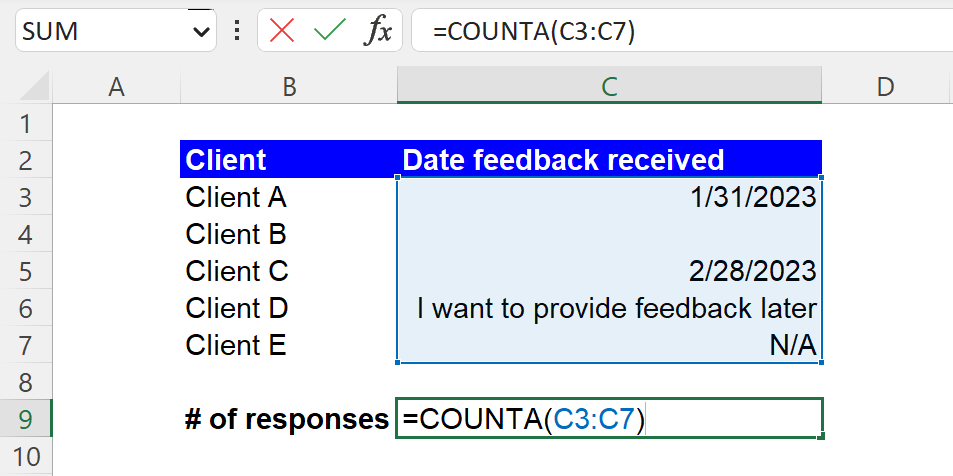Excel Formulas

# COUNTA Function in Excel: Explained

In this article, you will learn how to use the COUNT formula in Excel.

## What does the COUNTA formula in Excel?

The COUNTA formula counts the number of cells within a range that contain any type of data, including text, numbers, and errors. In other words, the COUNTA function counts the number of cells that are not empty.

## When do you use the COUNTA function in Excel?

The COUNTA function in Excel is beneficial when you want to count the number of non-empty cells in a range of cells, regardless of the type of data they contain (text, numbers, dates, etc.). This formula is helpful in various scenarios, such as:

1. Counting the number of entries in a database or list
2. Calculating the number of people who responded to a survey
3. Computing the number of cells that contain data in a specific column or row

## How to use the COUNTA formula in Excel?

The general syntax of the COUNTA function is as follows:

``=COUNTA(value1, [value2], ...)``

value1: This argument is a manual input, a cell, or a range that contains a value(s) you want to test.

value2: You can add other values to be tested up to 255 arguments.

Note 1: If a cell tested contains a formula returning an empty string, the COUNTA formula considers the cell as non-empty and thus counts it.

Note 2: If you want to count the number of cells containing only numeric values, use the COUNT formula instead.

Note 3: If you need to count the number of cells that meets one or more conditions, insert the COUNTIF or COUNTIFS functions.

Assume you have a dataset shown in the screenshot below, containing customer responses, if any, and a blank cell, if not, in the range of C3:C7. You want to compute how many pieces of feedback (or responses) you have received so far. The COUNTA function in cell C9 can be as follows:

``=COUNTA(C3:C7)``

The formula returns 4, because there are four non-empty cells in the selected range.How to use the COUNTA formula in Excel with an example

## What is the difference between the COUNTA and COUNTIFS functions in Excel?

The difference between the COUNTA and COUNTIFS functions in Excel is that the COUNTA function counts the number of non-empty cells within a range. In contrast, the COUNTIFS function calculates the number of cells within a range that meet one or more criteria. The COUNTIFS formula allows you to count cells based on specific conditions, such as counting the number of cells that contain a particular value, a number greater than a specific value, or a text string.

## Analyze your live financial data in a snap in Google Sheets

Are you learning this formula to visualize financial data, build a financial model, or conduct financial analysis? In that case, LiveFlow may help you automate manual workflows, update numbers in real-time, and save time. You can access various financial templates on our website, from the simple Income Statement to Multi-Currency Consolidated Financial Statement. Are you interested in this product but are an Excel user? That’s not a problem at all. You can connect Google Sheets to Excel quickly.